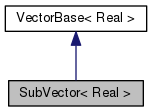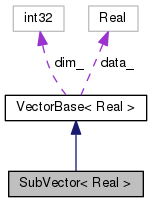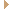SubVector< Real > Class Template Reference

Represents a non-allocating general vector which can be defined as a sub-vector of higher-level vector [or as the row of a matrix]. More...

`#include <kaldi-vector.h>`

Inheritance diagram for SubVector< Real >:[legend]
Collaboration diagram for SubVector< Real >:[legend]

## Public Member Functions

SubVector (const VectorBase< Real > &t, const MatrixIndexT origin, const MatrixIndexT length)
Constructor from a Vector or SubVector. More...

SubVector (const PackedMatrix< Real > &M)
This constructor initializes the vector to point at the contents of this packed matrix (SpMatrix or TpMatrix). More...

SubVector (const SubVector &other)
Copy constructor. More...

SubVector (const Real *data, MatrixIndexT length)
Constructor from a pointer to memory and a length. More...

SubVector (const MatrixBase< Real > &matrix, MatrixIndexT row)
This operation does not preserve const-ness, so be careful. More...

~SubVector ()
Destructor (does nothing; no pointers are owned here). More...Public Member Functions inherited from VectorBase< Real >
void SetZero ()
Set vector to all zeros. More...

bool IsZero (Real cutoff=1.0e-06) const
Returns true if matrix is all zeros. More...

void Set (Real f)
Set all members of a vector to a specified value. More...

void SetRandn ()
Set vector to random normally-distributed noise. More...

void SetRandUniform ()
Sets to numbers uniformly distributed on (0,1) More...

MatrixIndexT RandCategorical () const
This function returns a random index into this vector, chosen with probability proportional to the corresponding element. More...

MatrixIndexT Dim () const
Returns the dimension of the vector. More...

MatrixIndexT SizeInBytes () const
Returns the size in memory of the vector, in bytes. More...

Real * Data ()
Returns a pointer to the start of the vector's data. More...

const Real * Data () const
Returns a pointer to the start of the vector's data (const). More...

Real operator() (MatrixIndexT i) const
Indexing operator (const). More...

Real & operator() (MatrixIndexT i)
Indexing operator (non-const). More...

SubVector< Real > Range (const MatrixIndexT o, const MatrixIndexT l)
Returns a sub-vector of a vector (a range of elements). More...

const SubVector< Real > Range (const MatrixIndexT o, const MatrixIndexT l) const
Returns a const sub-vector of a vector (a range of elements). More...

void CopyFromVec (const VectorBase< Real > &v)
Copy data from another vector (must match own size). More...

template<typename OtherReal >
void CopyFromPacked (const PackedMatrix< OtherReal > &M)
Copy data from a SpMatrix or TpMatrix (must match own size). More...

template<typename OtherReal >
void CopyFromVec (const VectorBase< OtherReal > &v)
Copy data from another vector of different type (double vs. float) More...

template<typename OtherReal >
void CopyFromVec (const CuVectorBase< OtherReal > &v)
Copy from CuVector. This is defined in ../cudamatrix/cu-vector.h. More...

void Floor (const VectorBase< Real > &v, Real floor_val, MatrixIndexT *floored_count=nullptr)
Applies floor to all elements. More...

void Ceiling (const VectorBase< Real > &v, Real ceil_val, MatrixIndexT *ceiled_count=nullptr)
Applies ceiling to all elements. More...

void Pow (const VectorBase< Real > &v, Real power)

void ApplyLog ()
Apply natural log to all elements. More...

void ApplyLogAndCopy (const VectorBase< Real > &v)
Apply natural log to another vector and put result in *this. More...

void ApplyExp ()
Apply exponential to each value in vector. More...

void ApplyAbs ()
Take absolute value of each of the elements. More...

void ApplyFloor (Real floor_val, MatrixIndexT *floored_count=nullptr)
Applies floor to all elements. More...

void ApplyCeiling (Real ceil_val, MatrixIndexT *ceiled_count=nullptr)
Applies ceiling to all elements. More...

MatrixIndexT ApplyFloor (const VectorBase< Real > &floor_vec)
Applies floor to all elements. Returns number of elements floored. More...

Real ApplySoftMax ()
Apply soft-max to vector and return normalizer (log sum of exponentials). More...

Real ApplyLogSoftMax ()
Applies log soft-max to vector and returns normalizer (log sum of exponentials). More...

void Tanh (const VectorBase< Real > &src)
Sets each element of *this to the tanh of the corresponding element of "src". More...

void Sigmoid (const VectorBase< Real > &src)
Sets each element of *this to the sigmoid function of the corresponding element of "src". More...

void ApplyPow (Real power)
Take all elements of vector to a power. More...

void ApplyPowAbs (Real power, bool include_sign=false)
Take the absolute value of all elements of a vector to a power. More...

Real Norm (Real p) const
Compute the p-th norm of the vector. More...

bool ApproxEqual (const VectorBase< Real > &other, float tol=0.01) const
Returns true if ((*this)-other).Norm(2.0) <= tol * (*this).Norm(2.0). More...

void InvertElements ()
Invert all elements. More...

template<typename OtherReal >
void AddVec (const Real alpha, const VectorBase< OtherReal > &v)
Add vector : *this = *this + alpha * rv (with casting between floats and doubles) More...

void AddVec2 (const Real alpha, const VectorBase< Real > &v)
Add vector : *this = *this + alpha * rv^2 [element-wise squaring]. More...

template<typename OtherReal >
void AddVec2 (const Real alpha, const VectorBase< OtherReal > &v)
Add vector : *this = *this + alpha * rv^2 [element-wise squaring], with casting between floats and doubles. More...

void AddMatVec (const Real alpha, const MatrixBase< Real > &M, const MatrixTransposeType trans, const VectorBase< Real > &v, const Real beta)
Add matrix times vector : this <– beta*this + alpha*M*v. More...

void AddMatSvec (const Real alpha, const MatrixBase< Real > &M, const MatrixTransposeType trans, const VectorBase< Real > &v, const Real beta)
This is as AddMatVec, except optimized for where v contains a lot of zeros. More...

void AddSpVec (const Real alpha, const SpMatrix< Real > &M, const VectorBase< Real > &v, const Real beta)
Add symmetric positive definite matrix times vector: this <– beta*this + alpha*M*v. More...

void AddTpVec (const Real alpha, const TpMatrix< Real > &M, const MatrixTransposeType trans, const VectorBase< Real > &v, const Real beta)
Add triangular matrix times vector: this <– beta*this + alpha*M*v. More...

void ReplaceValue (Real orig, Real changed)
Set each element to y = (x == orig ? changed : x). More...

void MulElements (const VectorBase< Real > &v)
Multiply element-by-element by another vector. More...

template<typename OtherReal >
void MulElements (const VectorBase< OtherReal > &v)
Multiply element-by-element by another vector of different type. More...

void DivElements (const VectorBase< Real > &v)
Divide element-by-element by a vector. More...

template<typename OtherReal >
void DivElements (const VectorBase< OtherReal > &v)
Divide element-by-element by a vector of different type. More...

Add a constant to each element of a vector. More...

void AddVecVec (Real alpha, const VectorBase< Real > &v, const VectorBase< Real > &r, Real beta)
Add element-by-element product of vectors: More...

void AddVecDivVec (Real alpha, const VectorBase< Real > &v, const VectorBase< Real > &r, Real beta)
Add element-by-element quotient of two vectors. More...

void Scale (Real alpha)
Multiplies all elements by this constant. More...

void MulTp (const TpMatrix< Real > &M, const MatrixTransposeType trans)
Multiplies this vector by lower-triangular matrix: *this <– *this *M. More...

void Solve (const TpMatrix< Real > &M, const MatrixTransposeType trans)
If trans == kNoTrans, solves M x = b, where b is the value of *this at input and x is the value of *this at output. More...

void CopyRowsFromMat (const MatrixBase< Real > &M)
Performs a row stack of the matrix M. More...

template<typename OtherReal >
void CopyRowsFromMat (const MatrixBase< OtherReal > &M)

void CopyRowsFromMat (const CuMatrixBase< Real > &M)
The following is implemented in ../cudamatrix/cu-matrix.cc. More...

void CopyColsFromMat (const MatrixBase< Real > &M)
Performs a column stack of the matrix M. More...

void CopyRowFromMat (const MatrixBase< Real > &M, MatrixIndexT row)
Extracts a row of the matrix M. More...

template<typename OtherReal >
void CopyRowFromMat (const MatrixBase< OtherReal > &M, MatrixIndexT row)
Extracts a row of the matrix M with type conversion. More...

template<typename OtherReal >
void CopyRowFromSp (const SpMatrix< OtherReal > &S, MatrixIndexT row)
Extracts a row of the symmetric matrix S. More...

template<typename OtherReal >
void CopyColFromMat (const MatrixBase< OtherReal > &M, MatrixIndexT col)
Extracts a column of the matrix M. More...

void CopyDiagFromMat (const MatrixBase< Real > &M)
Extracts the diagonal of the matrix M. More...

void CopyDiagFromPacked (const PackedMatrix< Real > &M)
Extracts the diagonal of a packed matrix M; works for Sp or Tp. More...

void CopyDiagFromSp (const SpMatrix< Real > &M)
Extracts the diagonal of a symmetric matrix. More...

void CopyDiagFromTp (const TpMatrix< Real > &M)
Extracts the diagonal of a triangular matrix. More...

Real Max () const
Returns the maximum value of any element, or -infinity for the empty vector. More...

Real Max (MatrixIndexT *index) const
Returns the maximum value of any element, and the associated index. More...

Real Min () const
Returns the minimum value of any element, or +infinity for the empty vector. More...

Real Min (MatrixIndexT *index) const
Returns the minimum value of any element, and the associated index. More...

Real Sum () const
Returns sum of the elements. More...

Real SumLog () const
Returns sum of the logs of the elements. More...

void AddRowSumMat (Real alpha, const MatrixBase< Real > &M, Real beta=1.0)
Does *this = alpha * (sum of rows of M) + beta * *this. More...

void AddColSumMat (Real alpha, const MatrixBase< Real > &M, Real beta=1.0)
Does *this = alpha * (sum of columns of M) + beta * *this. More...

void AddDiagMat2 (Real alpha, const MatrixBase< Real > &M, MatrixTransposeType trans=kNoTrans, Real beta=1.0)
Add the diagonal of a matrix times itself: *this = diag(M M^T) + beta * *this (if trans == kNoTrans), or *this = diag(M^T M) + beta * *this (if trans == kTrans). More...

void AddDiagMatMat (Real alpha, const MatrixBase< Real > &M, MatrixTransposeType transM, const MatrixBase< Real > &N, MatrixTransposeType transN, Real beta=1.0)
Add the diagonal of a matrix product: *this = diag(M N), assuming the "trans" arguments are both kNoTrans; for transpose arguments, it behaves as you would expect. More...

Real LogSumExp (Real prune=-1.0) const
Returns log(sum(exp())) without exp overflow If prune > 0.0, ignores terms less than the max - prune. More...

void Write (std::ostream &Out, bool binary) const
Writes to C++ stream (option to write in binary). More...

template<>
void AddVec (const float alpha, const VectorBase< float > &rv)

template<>
void AddVec (const double alpha, const VectorBase< double > &rv)

template<>
void AddVec (const float alpha, const VectorBase< float > &v)

template<>
void AddVec (const double alpha, const VectorBase< double > &v)

## Private Member Functions

SubVectoroperator= (const SubVector &other)
Disallow assignment operator. More...Protected Member Functions inherited from VectorBase< Real >
~VectorBase ()
Destructor; does not deallocate memory, this is handled by child classes. More...

VectorBase ()
Empty initializer, corresponds to vector of zero size. More...

void CopyFromPtr (const Real *Data, MatrixIndexT sz)
Load data into the vector: sz must match own size. More...

KALDI_DISALLOW_COPY_AND_ASSIGN (VectorBase)Protected Attributes inherited from VectorBase< Real >
Real * data_
data memory area More...

MatrixIndexT dim_
dimension of vector More...

## Detailed Description

### template<typename Real> class kaldi::SubVector< Real >

Represents a non-allocating general vector which can be defined as a sub-vector of higher-level vector [or as the row of a matrix].

Definition at line 501 of file kaldi-vector.h.

## ◆ SubVector() [1/5]

 SubVector ( const VectorBase< Real > & t, const MatrixIndexT origin, const MatrixIndexT length )
inline

Constructor from a Vector or SubVector.

SubVectors are not const-safe and it's very hard to make them so for now we just give up. This function contains const_cast.

Definition at line 506 of file kaldi-vector.h.

507  : VectorBase<Real>() {
508  // following assert equiv to origin>=0 && length>=0 &&
509  // origin+length <= rt.dim_
510  KALDI_ASSERT(static_cast<UnsignedMatrixIndexT>(origin)+
511  static_cast<UnsignedMatrixIndexT>(length) <=
512  static_cast<UnsignedMatrixIndexT>(t.Dim()));
513  this->data_ = const_cast<Real*> (t.Data()+origin);
514  this->dim_ = length;
515  }
MatrixIndexT dim_
dimension of vector
Definition: kaldi-vector.h:397
Real * data_
data memory area
Definition: kaldi-vector.h:395
#define KALDI_ASSERT(cond)
Definition: kaldi-error.h:185

## ◆ SubVector() [2/5]

 SubVector ( const PackedMatrix< Real > & M )
inline

This constructor initializes the vector to point at the contents of this packed matrix (SpMatrix or TpMatrix).

Definition at line 519 of file kaldi-vector.h.

519  {
520  this->data_ = const_cast<Real*> (M.Data());
521  this->dim_ = (M.NumRows()*(M.NumRows()+1))/2;
522  }
MatrixIndexT dim_
dimension of vector
Definition: kaldi-vector.h:397
Real * data_
data memory area
Definition: kaldi-vector.h:395

## ◆ SubVector() [3/5]

 SubVector ( const SubVector< Real > & other )
inline

Copy constructor.

Definition at line 525 of file kaldi-vector.h.

References VectorBase< Real >::data_, and VectorBase< Real >::dim_.

525  : VectorBase<Real> () {
526  // this copy constructor needed for Range() to work in base class.
527  this->data_ = other.data_;
528  this->dim_ = other.dim_;
529  }
MatrixIndexT dim_
dimension of vector
Definition: kaldi-vector.h:397
Real * data_
data memory area
Definition: kaldi-vector.h:395

## ◆ SubVector() [4/5]

 SubVector ( const Real * data, MatrixIndexT length )
inline

Constructor from a pointer to memory and a length.

Keeps a pointer to the data but does not take ownership (will never delete). Caution: this constructor enables you to evade const constraints.

Definition at line 534 of file kaldi-vector.h.

References VectorBase< Real >::data_, and VectorBase< Real >::dim_.

534  : VectorBase<Real> () {
535  this->data_ = const_cast<Real*>(data);
536  this->dim_ = length;
537  }
MatrixIndexT dim_
dimension of vector
Definition: kaldi-vector.h:397
Real * data_
data memory area
Definition: kaldi-vector.h:395

## ◆ SubVector() [5/5]

 SubVector ( const MatrixBase< Real > & matrix, MatrixIndexT row )
inline

This operation does not preserve const-ness, so be careful.

Definition at line 540 of file kaldi-vector.h.

540  {
541  this->data_ = const_cast<Real*>(matrix.RowData(row));
542  this->dim_ = matrix.NumCols();
543  }
MatrixIndexT dim_
dimension of vector
Definition: kaldi-vector.h:397
Real * data_
data memory area
Definition: kaldi-vector.h:395

## ◆ ~SubVector()

 ~SubVector ( )
inline

Destructor (does nothing; no pointers are owned here).

Definition at line 545 of file kaldi-vector.h.

## ◆ operator=()

 SubVector& operator= ( const SubVector< Real > & other )
inlineprivate

Disallow assignment operator.

Definition at line 549 of file kaldi-vector.h.

References kaldi::operator>>().

549 {}

The documentation for this class was generated from the following file: